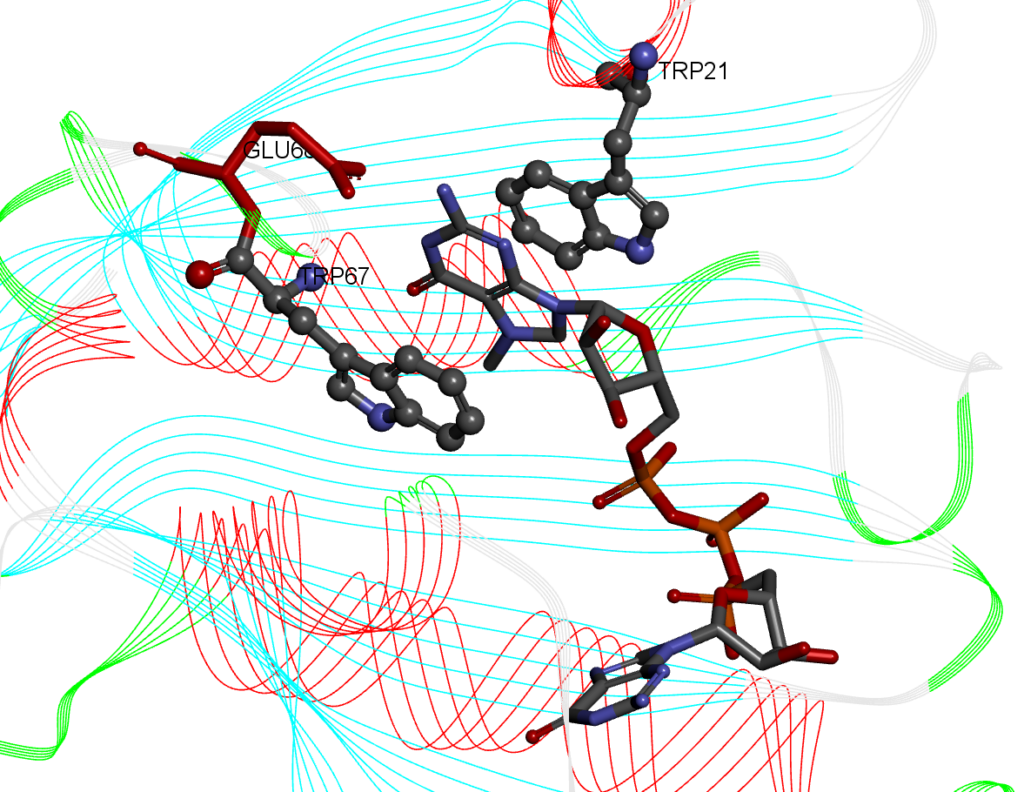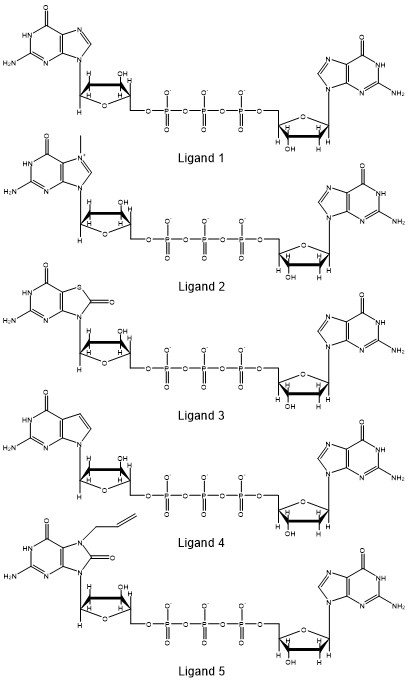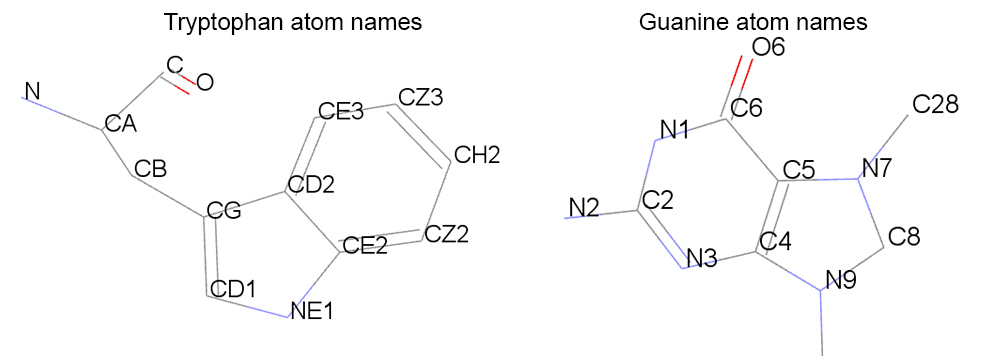# Measure the stacking percentage of a protein-ligand complex

You want to measure the stacking percentage between a protein and a ligand

For this recipe we will measure the stacking percentage between five ligands that have an aromatic planar component (guanine) and a protein.  The system we will use is the cocrystal Structure of the Messenger RNA 5′ Cap-binding Protein (eIF4E) bound to 7-methylGpppG (PDB: 1L8B). The 7-methylGpppG molecule interacts with two tryptohpan residues in the binding pocket making pi-pi stacking interactions between the 7-methylG part of the 7-methylGpppG ligand and two tryptohan residues of the protein (in the crystal structures, the residues are TRP56 and TRP102. In our model the TRP residues are 21 and 67) as shown below:An example of a simulation with ligand 2 and the eIF4E receptor is shown below:

The stacking percentage will provide information on how the different ligands interact with the protein. We will analyze the stacking percentage of the interaction between the eIF4E protein and  five different variants of GpppG from molecular dynamics simulations using CPPTRAJ. The ligands are analogs of guanine and we want to study how the analogs affect the interaction in the binding pocket. One of the many analysis we can perform is to measure how the different analogs affect the stacking conformation. The analogs are:Ligand 2 is the methylated guanine molecule from the crystal structure. There are several approaches to evaluate if two planar molecules are forming stacking interactions, for our example, we  will measure the distance between the tryptophan molecule (TRP21) and ligand, and the angle between the normal (perpendicular) vector between the tryptophan and the ligand. A similar approach has been used by Hayatsahi et. al. to study the stacking percentage of dinucleotides. With this approach, we will have a distance measure and an angle measure. From this data we can count the number of frames that the data fit within a certain threshold that we will set.

The threshold that we will use to evaluate if a tryptophan is forming a stacking interaction with the ligand will consist in the distance between the center of mass (COM) of the aromatic part of the tryptohan (atoms: CG,CD1,NE1,CE2,CZ2,CH2,CZ3,CE3,CD2) and the aromatic ring of the guanine residue from the ligand (atoms: N1,C2,N3,C4,C5,C6,N7,C8,N9).For our example we will consider if the ligand and the tryptophan molecules are stacked if:

• The minimum distance between the center of mass (COM) is 5 Å and,
• The angles between the normal to two bases are between 0°and 45°.

If the values of COM and the angle fall into these two criteria, we will assume that the ligand is forming a stacking interaction with the tryptophan molecules in the binding pocket. First we will study the stacking interaction between TRP21-ligand and then TRP67-ligand. Using CPPTRAJ, we can use the distance command to measure the distance between the tryptophan and the ligands, and the vector command to obtain the coordinates of the normal vector for each molecule. Using the vectormath command, we can then measure the angle between the normal vectors for each molecule. The example will assume that you have pre-processed the trajectories (deleted the waters and re-orient the solute molecules using autoimage, see this recipe for an example). The CPPTRAJ input looks like this:

```### Read the topology file
parm nowater.prmtop

### Read the trajectory file (start from 1st frame until the last frame, read every 1 frame)
trajin nowater.nc 1 last 1

### Use the distance command between residues 21 and 183
### Use only the atoms that are in the rings
### Name the dataset as COM
distance COM :21@CG,CD1,NE1,CE2,CZ2,CH2,CZ3,CE3,CD2 :183@N1,C2,N3,C4,C5,C6,N7,C8,N9

### Use the vector command to calculate the normal vector for each residue
### Name the dataset as TRP21 for residue 21 and LIG for residue 183
vector TRP21 corrplane :21@CG,CD1,NE1,CE2,CZ2,CH2,CZ3,CE3,CD2
vector LIG corrplane :183@N1,C2,N3,C4,C5,C6,N7,C8,N9

### Calculate the angle between the vectors of residue 21 and 183
### Name the dataset as ANGLE
vectormath name ANGLE vec1 TRP21 vec2 LIG dotangle

### Run the calculations to create the datasets
run

### Use the writedata command to write the results
### Name the results file as com-angle.dat
writedata com-angle.dat COM ANGLE```

The CPPTRAJ script will read the topology and the trajectory file, then will measure the distance between residues 21 and 183 based on the atom names that are involved in the aromatic rings and save the distance information in a dataset with the name COM. Then it will calculate the normal vector using the `corrplane` keyword of each of the residues, based in the atoms of the planar rings and save the information in the dataset with name TRP21 and LIG for tryptophan 21 and the ligand (which is residue id 183) respectively. Using the created datasets TRP21 and LIG, which have vector information, the vectormath command will calculate the dot product and write the results in the ANGLE dataset. To populate the datasets, we need to execute the analysis first with the run keyword. Using the writedata command we can write the contents of the COM and ANGLE datasets to the `com-angle.dat` file. The file looks like:

```#Frame COM     ANGLE
1      3.3496  4.9660
2      3.6310  1.0175
3      3.6409  13.9994
4      3.9028  19.5007
5      3.9050  24.9501
6      4.2884  29.6259
7      5.0748  46.5155
8      4.2550  21.8009
9      5.5804  65.3985.........```

The first column is the frame number, the second column is the distance between the atoms of the planar rings of residues 21 and 183 and the third column is the dot product result, calculated from the normal vectors from the vector command for residues 21 and 183. We can then use a script to calculate the % of interactions that meet the distance and angle values that will meet our conditions to consider the interaction between the ligand and the tryptophan as stacked. For every frame, the distance has to be equal or less than 5 and the angle value has to be between 0 and 45. As an example, we can consider the following python script:

``````#!/usr/bin/env python3
count = -1
stacked = 0
with open('com-angle.dat') as data:
next(data)
for line in data:
count += 1
frame,distance,angle = line.split()
dis=float(distance)
ang=float(angle)
if (ang >= 0 and ang <= 45) and (dis <= 5):
stacked += 1
print(dis,ang)
print("Total frames:",count)
print("Total stacked frames:",stacked)
TOTALSTACKED=(stacked*100/count)
print("Total stacked %:",TOTALSTACKED)``````

This will open our `com-angle.dat` file from CPPTRAJ, read every line and increment the count variable by 1 when the data meets the specified values. We can then obtain the % of stacking that each ligand has from our molecular dynamics simulations: